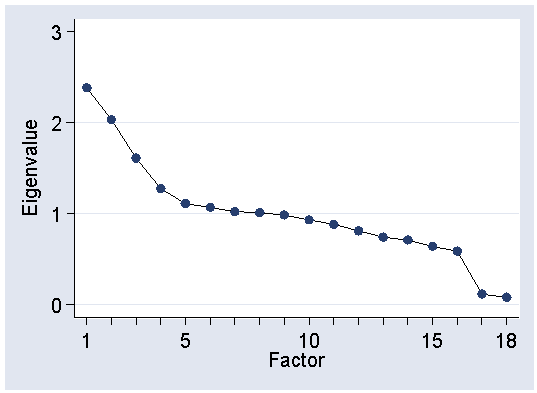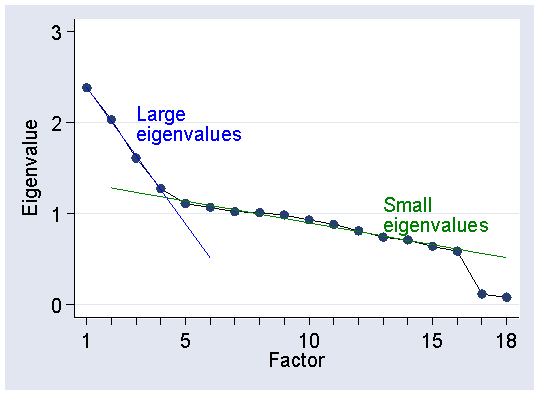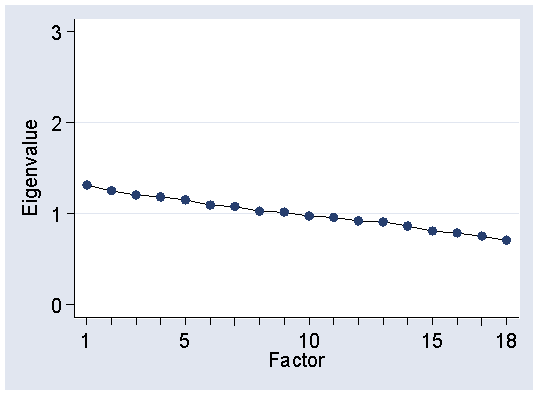# Exercise: Active listening scale, answer 2

Question 2: What is a scree test and for what is it used?

The scree plot, rather than test, is used to decide how many factors are present. It uses something called the eigenvalues, related to the proportion of total variance explained by the factor. The first part of the factor analysis produces as many factors as there are variables, all uncorrelated and each with an eigenvalue. The sum of the eigenvalues is equal to the number of variables. The factors are ordered by their eigenvalues. We then plot the eigenvalue against the factor number:This is called a scree plot because it resembles the scree formed by fallen rocks at the bottom of an escarpment. If there are factors which explain some of the variation, we should be able to see two distinct lines on the plot: a steep line where there are real factors and a shallow line where the factors represent only random variation.

We can draw these lines on the graph:In this picture the steep line goes through 4 points and there are four factors, represented by the four largest eigenvalues.

If we do not have any real factors, there should be only one line apparent. The folowing graph was generated by factor analysis of 18 variables generated from independent random numbers. They have no relationships other than those arising by chance and hence no factors. There is only one line on the plot.Back to question.